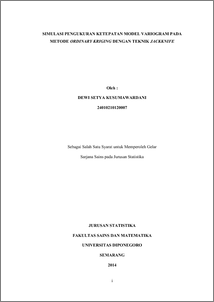# SIMULASI PENGUKURAN KETEPATAN MODEL VARIOGRAM PADA METODE ORDINARY KRIGING DENGAN TEKNIK JACKKNIFE

KUSUMAWARDANI, DEWI SETYA (2014) SIMULASI PENGUKURAN KETEPATAN MODEL VARIOGRAM PADA METODE ORDINARY KRIGING DENGAN TEKNIK JACKKNIFE. Undergraduate thesis, FSM Undip.Preview
PDF
702Kb

## Abstract

Kriging is the one of geostatistical data analysis and it is useful for estimate values which represents a sample point unknown based on sample point known in the surrounding. In the ordinary kriging, estimation of the value at certain point done by observing similiar data at the other location, at every point of an unknown value, then it will be estimate by using weighted linier combination of the available samples. Data in this case study is a random data that generated based on variogram model and it defined as iron content data (%), variogram that used to generate the data is spherical and eksponential. Estimated value is obtained through a system of Ordinary Kriging using Jackknife technique. The accuracy of the spherical and exponential variogram model is calculated based on the midpoint error absolute percentage error ( Mean Absolute Percentage Error ). Percentage error based on result of calculations for spherical variogram is 0.0417%, while the percentage error for the exponential variogram models is 0.0776 %. Both the MAPE values less than 10%, thus it can be concluded that jackknife tecnique can be used to determined estimated value from ordinary kriging system from the spherical and exponential variogram models. Keyword: ordinary kriging, variogram, jackknife, MAPE.

Item Type: Thesis (Undergraduate) H Social Sciences > HA Statistics Faculty of Science and Mathematics > Department of Statistics 43506 Mr Hasbi Yasin 19 Aug 2014 16:18 19 Aug 2014 16:18

Repository Staff Only: item control page